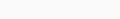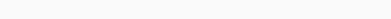# Coursera machine learning week 3 Quiz answer Logistic Regression | Andrew Ng

In this article, you will find Coursera machine learning week 3 Quiz answer Logistic Regression | Andrew NG. Use “Ctrl+F” To Find Any Questions or Answers. For Mobile Users, You Just Need To Click On Three dots In Your Browser & You Will Get A “Find” Option There. Use These Options to Get Any Random Questions Answer.
Try to solve all the assignments by yourself first, but if you get stuck somewhere then feel free to browse the code. Don’t just copy-paste the code for the sake of completion.  Even if you copy the code, make sure you understand the code first.

### Coursera machine learning week 3 Quiz answer Logistic Regression | Andrew NG

1. Suppose that you have trained a logistic regression classifier, and it outputs on a new example a prediction= 0.2. This means (check all that apply):

•  Our estimate for P(y = 1|x; θ) is 0.8.

h(x) gives P(y=1|x; θ), not 1 – P(y=1|x; θ)

•  Our estimate for P(y = 0|x; θ) is 0.8.

Since we must have P(y=0|x;θ) = 1 – P(y=1|x; θ), the former is
1 – 0.2 = 0.8.

•  Our estimate for P(y = 1|x; θ) is 0.2.

h(x) is precisely P(y=1|x; θ), so each is 0.2.

•  Our estimate for P(y = 0|x; θ) is 0.2.

h(x) is P(y=1|x; θ), not P(y=0|x; θ)

2. Suppose you have the following training set, and fit a logistic regression classifier .Which of the following are true? Check all that apply.

Sharing Is Caring